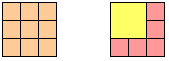#### You may also like### Square Areas

Can you work out the area of the inner square and give an explanation of how you did it?### 2001 Spatial Oddity

With one cut a piece of card 16 cm by 9 cm can be made into two pieces which can be rearranged to form a square 12 cm by 12 cm. Explain how this can be done.### Tetra Square

ABCD is a regular tetrahedron and the points P, Q, R and S are the midpoints of the edges AB, BD, CD and CA. Prove that PQRS is a square.

# Dissect

##### Age 11 to 14 Challenge Level:

It is possible to dissect any square drawn on a grid into smaller squares, by cutting along grid lines.For example a 3 by 3 square can be dissected into either 9 smaller squares or 6 squares:

• What is the minimum number of squares a 13 by 13 square can be dissected into?

• What is the smallest size square which can be dissected into squares which are all different sizes?